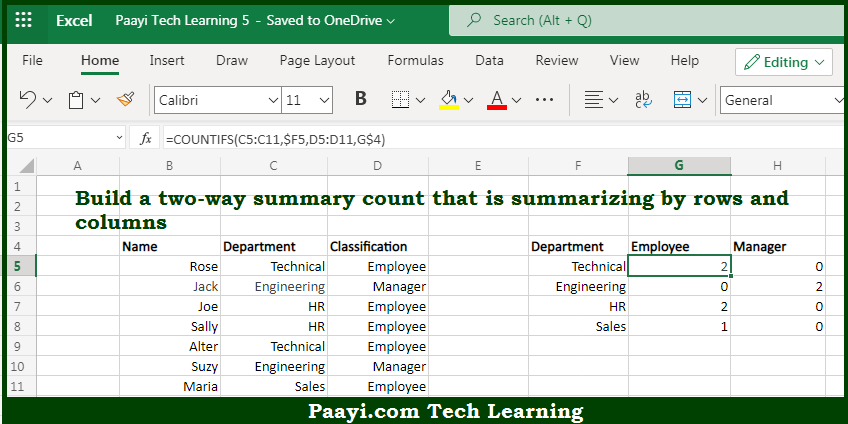# Learn How to Two Way Summary COUNT With COUNTIFS in Microsoft Excel

Written by | 0 Comments | 547 Views

In this article, you will learn how to COUNT various things in Microsoft Excel using a single/combination(s) of functions. You will also know how to Two Way Summary COUNT With COUNTIFS and see the generic formula.

Two Way Summary COUNT With COUNTIFS in Microsoft Excel

The main purpose of this formula is to build a two-way summary count that is summarizing by rows and columns. Here we will learn how to create a two-way summary count with COUNTIFS in Microsoft Excel. That implies, with the help of a formula based on the COUNTIFS function you can able to build a two-way summary count that is summarizing by rows and columns. So, with the help of this formula, you can able to create a two-way summary count with COUNTIFS in Microsoft Excel.

General Formula to Summary COUNT With COUNTIFS

=COUNTIFS(rng1,crit1,rng2,crit2)

The Explanation for the Summary COUNT With COUNTIFSSo we know that with the help of the given formula above you can able to build a two-way summary count that is summarizing by rows and columns. Here we will learn how to create a two-way summary count with COUNTIFS in Microsoft Excel. As we know that the COUNTIFS function is specially designed to count things based on more than one criteria. In the example provided here, the target is to build a summary table first that contains one set of criteria in the left-most column, and the second set of criteria as column headings. So, with the help of this formula, you can able to create a two-way summary count with COUNTIFS in Microsoft Excel.